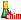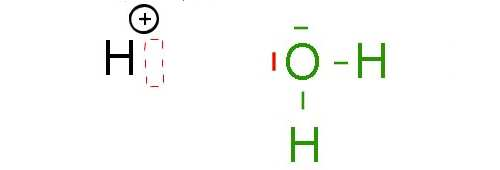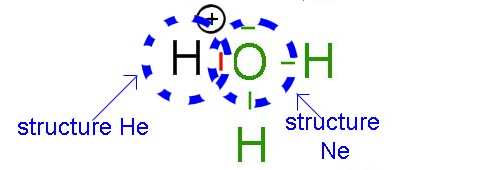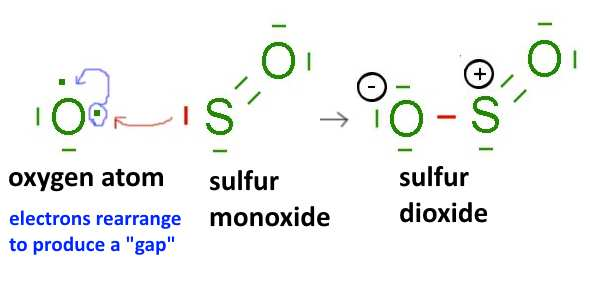Search:

# Dative (semipolar, coordinate) bonds

## Lewis model

In the hydronium (hydroxonium) ion $H_3O^+$, the oxygen atom O makes 3 bonds with hydrogen atoms H. The simple Lewis model of the covalent bond (as many covalent bonds than lone electrons) must be expanded to take into account of that kind of ions:Here a hydrogen ion and a water molecule. The hydrogen ion has room for two electrons in its last layer. Indeed, the last layer of the $H^+$ ion is empty, but can accommodate a maximum of 2 electrons. It is said that the $H^+$ ion has an electron gap On the other side, the oxygen atom in the water molecule has (at least) one electron pair on the last layer. The "deal" is now as follows:By combining with one doublet of the oxygen atom, $H$ acquires the stable helium structure and $O$ the same structure as the noble gas neon!It should anyway be realized, that by sharing two of its electrons with hydrogen, the O atom loses on average one electron, while H wins one, so that O, formerly neutral acquires a positive charge while H, positively charged at the beginning is now neutral:A dative bond can form between a chemical species with an electronic gap and a species with an electron pair. The first species on average gains one electron and its charge decreases by one, the second loses one electron and its charge increases by one

Here different cases of octet violators (electron deficient species, presenting "electron gaps"): - The hydrogen ion , for example:- Compounds of earth metals (third family column of the periodic table, boron group): $B$, $Al$ ..., for example:- Atoms of chalcogens (sixth family column of the periodic table, oxygen group): $O$, $S$, .... after rearrangement of their outer electrons, for example: